# Averages and Correlations

This document defines notation that I use for indicating “average” values, and also defines a particular type of “correlation” factor that I use.

## Overview

I this section, I’ll try to summarize the minimum you need to know about averages in order to to be able to follow my analyses.

• I denote the average of a quantity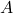by the notation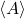.
• In the context of my climate analyses,generally indicates a global average (i.e., an average over the surface of the globe) within some time window.
• I sometimes express things in terms of the weighted average ofrelative to a weight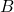, which I denote as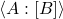. The value of this weighted average is always within the range of variation of, i.e., between the minimum and maximum values of.
• I sometimes express things in terms of the multiplicative correlation ofand, which I denote as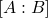. This is a dimensionless quantity. Whenandare uncorrelated,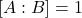. However, in general, it could be larger or smaller than 1. The notation can be extended to multiple variables, as in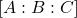, which denotes the correlation of,, and.

These values are related by several identities, which I often use to transform the way a quantity is expressed:

1.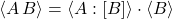2.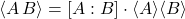3.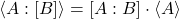(I use the notation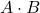to indicate multiplication when I want to make the factors more visually distinct.)

If you are reading one of my analyses, taking in the above information may be enough to allow you to follow the analysis and understand the results. If not, then more detailed information is provided below.

## Averages

### Average

Ifis a discrete quantity and there are a finite number of instances of, then the average value of, denotedis given by:

(1)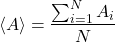Ifis a continuous quantity which varies with respect to a variable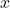, then the value ofaveraged overis given by:

(2)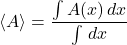Averages are “linear,” meaning they have behavior in a simple way with respect to addition and multiplication by a constant. In particular, if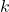is a constant, then:

(3)(4)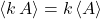### Average over more than one variable

One can average over different variables. When I want to distinguish averages with respect to different variables, I denote the average ofwith relative to the variableby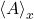. When averaging over multiple variables, it doesn’t matter in which order averaging is done:

(5)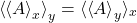### Weighted average

The weighted average ofwith respect tois defined by:

(6)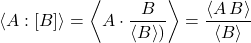When working with averages of quantities that are the result of multiplying several factors together, using weighted averages can make expressions work out more neatly.

For example, when talking about Earth’s albedo, the global albedo value quoted typically reflects the average of local albedo values, as weighted by the intensity of received sunlight. This makes the math work out simply: To determine the total fraction of sunlight reflected, it’s only necessary to multiply the total sunlight incident on the Earth by the global albedo value. (If one used an unweighted average of local albedo values in that calculation, that would yield an incorrect answer.)

Ifhas minimum value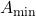and maximum value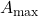, then, providedeverywhere,will always be between these two limits:

(7)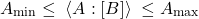## Multiplicative Correlations

When people talk about the “correlation” of two variables, they are typically talking about what could be referred to as an “additive” correlation. Such a “correlation” ofandinvolves calculating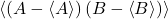. The relationship between each variable’s additive deviation from its mean (average) vale.

Rather than focusing on additive correlations, I’d like to consider each variable’s multiplicative deviation from its mean value. I denote the multiplicative correlation ofand(or the multiplicative correlation factor forand) aswhere this is defined by:

(8)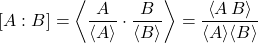This can be generalized to more variables:

(9)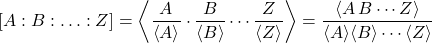Variablesandare said to be “uncorrelated” if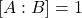.

Writing formulas using multiplicative correlation factors allows one to clearly distinguish what depends on the average values of individual variables, and what depends on relationships between variables (as characterized by their correlation).

If the variablesandsatisfies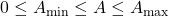and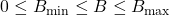, then their multiplicative correlation satisfies:

(10)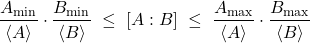Note: The terms I use, “additive correlation” and “multiplicative correlation,” might not match terminology used elsewhere.

## Identities

The definitions above lead to these identities, which will sometimes be used in my analysis:

(11)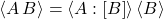(12)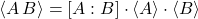(13)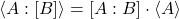## Averages for global climate analysis

### Typical averaging

In my analyses of climate, unless I indicate otherwise, averages should be interpreted as being averages over the surface of the globe within some time window:

(14)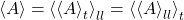where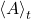is the average over a time window, and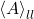is the average over latitude and longitude.

### Time average

When dealing with climate data, data is usually averaged over some time window, typically at least over a day, if not over a month or a year.

A time average can be defined relative to a series of discrete time windows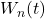or relative to a sliding time window,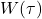.

#### Time average over a discrete time window

The average of a variable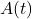relative to a discrete time windowis defined as:

(15)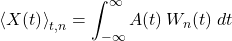where the time window is normalized so that:

(16)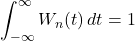#### Time average over a sliding time window

The average of a variablerelative to a sliding time windowis defined as:

(17)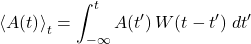where the time widows is normalized so that:

(18)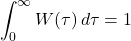### Average over latitude and longitude

The average ofover latitude and longitude is made up of the averages over those two variables:

(19)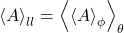#### Average over longitude: zonal average

If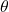and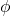are latitude and longitude (expressed in radians), then the average ofover longitude is:

(20)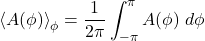In the context of climate, an average like this that eliminates longitude and leaves only latitude is referred to as a “zonal” average.

#### Average over latitude

For simple applications, the average ofover latitude may be computed as:

(21)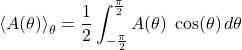However, that formula assumes the planet is a sphere. More accurate work must take into account the actual shape of a planet. Earth is more accurately treated as an oblate-spheroid. The NASA CERES project provides information on how to apply geodetic weighting to compute accurate global averages.